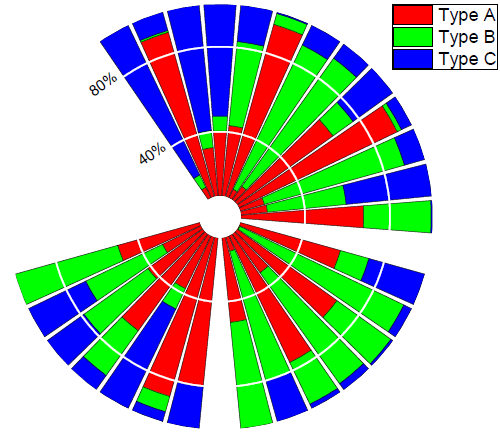Skip Navigation Links.

### Stacked polar column chartDescription: The basic graph type here is a "Theta (X) r(Y)" polar plot, meaning that the angular data are contained in a single X column and the radius data are contained in a single Y column. In this graph, after making a standard polar plot, we open the Plot Details dialog box and change the Plot Type to Column/Bar to make a polar column plot.

Tutorial: To see how this graph was created, view an online tutorial.